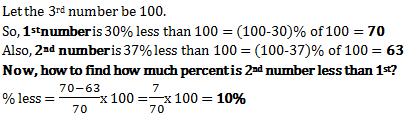# Percentage - Quantitative Aptitude (MCQ) questions for Q. 29188

Q.  Two numbers are less than a third number by 30% and 37% respectively. How much percent is the second number less than the first?
- Published on 05 Apr 17

a. 7%
b. 10%
c. 4%
d. 3%